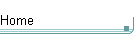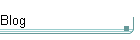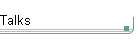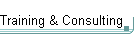GotW #12On theblogNovember 4: Other Concurrency Sessions at PDC November 3 October 26: Hoare on Testing October 23This is the original GotW problem and solution substantially as posted to Usenet. See the book Exceptional C++ (Addison-Wesley, 2000) for the most current solutions to GotW issues #1-30. The solutions in the book have been revised and expanded since their initial appearance in GotW. The book versions also incorporate corrections, new material, and conformance to the final ANSI/ISO C++ standard.

## Control FlowDifficulty: 6 / 10

How well do you really know the order in which C++ code is executed? Test your knowledge against this problem.### Problem

"The devil is in the details." Point out as many problems as possible in the following (somewhat contrived) code, focusing on those related to control flow.

```    #include <cassert>
#include <iostream>
#include <typeinfo>
#include <string>
using namespace std;

//  The following lines come from other header files.
//
char* itoa(int value, char* workArea, int radix );
extern int fileIdCounter;

//  Helpers to automate class invariant checking.
//
template<class T>
inline void AAssert( T& p ) {
static int localFileId = ++fileIdCounter;
if( !p.Invariant() ) {
cerr << "Invariant failed: file " << localFileId
<< ", " << typeid(p).name()
<< " at " << static_cast<void*>(&p) << endl;
assert( false );
}
}

template<class T>
class AInvariant {
public:
AInvariant( T& p ) : p_(p) { AAssert( p_ ); }
~AInvariant()              { AAssert( p_ ); }
private:
T& p_;
};
#define AINVARIANT_GUARD AInvariant<AIType>  \
invariantChecker( *this )

//-------------------------------------------------
class Array : private ArrayBase, public Container {
typedef Array AIType;
public:
Array( size_t startingSize = 10 )
: Container( startingSize ),
ArrayBase( Container::GetType() ),
used_(0),
size_(startingSize),
buffer_(new char[size_])
{
AINVARIANT_GUARD;
}

void Resize( size_t newSize ) {
AINVARIANT_GUARD;
char* oldBuffer = buffer_;
buffer_ = new char[newSize];
memset( buffer_, ' ', newSize );
copy( oldBuffer, oldBuffer+min(size_,newSize),
buffer_ );
delete[] oldBuffer;
size_ = newSize;
}

string PrintSizes() {
AINVARIANT_GUARD;
char buf;
return string("size = ") + itoa(size_,buf,10) +
", used = " + itoa(used_,buf,10);
}

bool Invariant() {
if( used_ > 0.9*size_ ) Resize( 2*size_ );
return used_ <= size_;
}
private:
char*  buffer_;
size_t used_, size_;
};

int f( int& x, int y = x ) { return x += y; }
int g( int& x )            { return x /= 2; }

int main( int, char*[] ) {
int i = 42;
cout << "f(" << i << ") = " << f(i) << ", "
<< "g(" << i << ") = " << g(i) << endl;
Array a(20);
cout << a.PrintSizes() << endl;
}```### Solution

"Lions and tigers and bears, oh my!"
-- Dorothy

```    #include <cassert>
#include <iostream>
#include <typeinfo>
#include <string>
using namespace std;

//  The following lines come from other header files.
//
char* itoa(int value, char* workArea, int radix );
extern int fileIdCounter;```

The presence of a global variable should already put us on the lookout for clients that might try to use it before it has been initialized. The order of initialization for global variables (including class statics) between translation units is undefined.

```    //  Helpers to automate class invariant checking.
//
template<class T>
inline void AAssert( T& p ) {
static int localFileId = ++fileIdCounter;```

Aha, here we have a case in point. If the compiler happens to initialize fileIdCounter before it initializes any AAssert<T>::localFileId, well and good. Otherwise, the value set will be based on whatever was in fileIDCounter's raw memory before it was initialized.

```        if( !p.Invariant() ) {
cerr << "Invariant failed: file " << localFileId
<< ", " << typeid(p).name()
<< " at " << static_cast<void*>(&p) << endl;
assert( false );
}
}

template<class T>
class AInvariant {
public:
AInvariant( T& p ) : p_(p) { AAssert( p_ ); }
~AInvariant()              { AAssert( p_ ); }
private:
T& p_;
};
#define AINVARIANT_GUARD AInvariant<AIType> \
invariantChecker( *this )```

These helpers are an interesting idea, where any client class that would like to automatically check its class invariants before and after function calls simply writes a typedef of AIType to itself, then writes "AINVARIANT_GUARD;" as the first line of member functions. Not entirely bad, in itself.

In the client code below, these ideas go unfortunately astray. The main reason for this is that AInvariant hides calls to assert(), which will be automatically removed by the compiler when the program is built in non-debug mode. As written, the following client code was likely written by a programmer who wasn't aware of this build dependency and the resulting change in side effects.

```    //-------------------------------------------------
class Array : private ArrayBase, public Container {
typedef Array AIType;
public:
Array( size_t startingSize = 10 )
: Container( startingSize ),
ArrayBase( Container::GetType() ),```

This constructor's initializer list has two potential errors. This first one is NOT necessarily an error, but was left in as a bit of a red herring:

1. If GetType() is a static member function, or a member function that does not use its 'this' pointer (i.e., uses no member data) and does not rely on any side effects of construction (e.g., static usage counts), then this is merely poor style but will run correctly.

2. Otherwise (mainly, if GetType() is a normal nonstatic member function), then we have a problem. Nonvirtual base classes are initialized in left-to-right order as they are declared, and so ArrayBase is initialized before Container. Unfortunately, that means we're trying to use a member of the not-yet-initialized Container subobject.

```            used_(0),
size_(startingSize),
buffer_(new char[size_])```

This second is indeed an error, since the variables will be actually initialized in the order in which they appear later in the class definition:

```            buffer_(new char[size_])
used_(0),
size_(startingSize),```

Writing it this way makes the error obvious. The call to new[] will make buffer an unpredictable size -- typically zero or something extremely large, depending on whether the compiler happens to initialize object memory to nulls before invoking constructors. At any rate, the initial allocation is unlikely to end up actually being for 'startingSize' bytes.

```        {
AINVARIANT_GUARD;
}```

Minor efficiency issue: Here the Invariant() function will be needlessly called twice, once during construction and again during destruction of the hidden temporary. This is a nit, though, and is unlikely to be a real issue.

```        void Resize( size_t newSize ) {
AINVARIANT_GUARD;
char* oldBuffer = buffer_;
buffer_ = new char[newSize];
memset( buffer_, ' ', newSize );
copy( oldBuffer, oldBuffer+min(size_,newSize),
buffer_ );
delete[] oldBuffer;
size_ = newSize;
}```

There is a serious control flow problem here. I didn't see anyone point it out (sorry if I missed anyone), which is good since I deliberately put it in to see if anyone would comment.

Before reading on, examine the function again to see if you can spot a (hint: pretty obvious) control flow problem.

* * * * * * * * * * * *

Answer: It's not exception-safe. If the call to new[] throws a bad_alloc exception, not only is the current object left in an invalid state, but the original buffer is leaked since all pointers to it are lost and so it can never be deleted.

The point of this function was to show how, so far, it seems that few if any programmers yet write exception-safe code as a matter of habit -- even after we recently had an extensively discussed GotW on exception safety!

```        string PrintSizes() {
AINVARIANT_GUARD;
char buf;
return string("size = ") + itoa(size_,buf,10) +
", used = " + itoa(used_,buf,10);
}```

The prototyped itoa() function uses the passed buffer as a scratch area. However, there's a control flow problem because there's no way to predict the order in which the expressions in the last line are evaluated, because the order in which function parameters are ordered is undefined and implementation-dependent. (Note that this would not be true for the BUILTIN operator+, but as soon as you provide your own the rules change to the rules for function calls.)

What the last line really amounts to is something like this, since + calls are still performed left-to-right:

```    return
operator+(
operator+(
operator+( string("size = "),
itoa(size_,buf,10) ) ,
", y = " ) ,
itoa(used_,buf,10) );```

Say that size_ is 10 and used_ is 5. Then if the outer operator+()'s first parameter is evaluated first, the output will be the correct "size = 10, used = 5" since the results of the first itoa() is used and stored in a temporary string before the second itoa() reuses the same buffer. If the outer operator+()'s second parameter is evaluated first (as it is, for example, under MSVC 4.x), the output will be the incorrect "size = 10, used = 10" since the outer itoa() is executed first and then the inner itoa() will clobber the results of the outer itoa() before either value is used.

```        bool Invariant() {
if( used_ > 0.9*size_ ) Resize( 2*size_ );
return used_ <= size_;```

The call to Resize() has two problems.

1. In this case, the program wouldn't work at all anyway, since if the condition is true then Resize() will be called, only to immediately call Invariant() again, which will find the condition still true and will call Resize() again, which... you get the idea.

2. What if, for efficiency, the writer of AAssert() decided to remove the error reporting and simply wrote "assert( p->Invariant() );"? Then this client code becomes deplorable style, because it puts code with side effects inside an assert() call. This means the program's behaviour will be different when compiled in debug mode than it is when compiled in release mode. Even without the first problem above, this would be bad because it means that Array objects will resize at different times depending on the build mode, which will make the testers' lives a living hell as they try to reproduce customer problems on a debug build that ends up having a different runtime memory image characteristics.

Bottom line: Never write code with side effects inside a call to assert() (or something that might be one), and always make sure your recursions really terminate.

```        }
private:
char*  buffer_;
size_t used_, size_;
};

int f( int& x, int y = x ) { return x += y; }```

The second parameter default isn't legal C++ at any rate, so this shouldn't compile under a conforming compiler (though some systems will take this). One reason this is bad is again that the compiler is free to choose the order in which it wants to evaluate the function parameters, so if this were allowed then y might be initialized before x.

```    int g( int& x )            { return x /= 2; }

int main( int, char*[] ) {
int i = 42;
cout << "f(" << i << ") = " << f(i) << ", "
<< "g(" << i << ") = " << g(i) << endl;```

Here we run into parameter evaluation ordering again. Since there's no telling the order in which f(i) or g(i) will be executed (or, for that matter, the ordering of the two bald evaluations of 'i' itself), the printed results can be quite incorrect. One example result is MSVC's "f(22) = 22, g(21) = 21", which means the compiler is likely evaluating all function arguments in order from right to left.

But isn't the result wrong? No, the compiler is right... and another compiler could print out something else and still be right, too, because the programmer is relying on something that is undefined in C++.

```        Array a(20);
cout << a.PrintSizes() << endl;
}```

Perhaps Dorothy wasn't quite right... the following might be closer:

"Parameters and globals and exceptions, oh my!"
-- Dorothy, after an intermediate C++ course